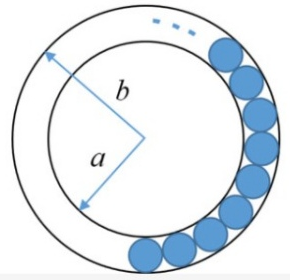GATE | GATE CS 2020 | Question 8

• Last Updated : 26 May, 2021

The figure below shows an annular ring with outer and inner as b and a, respectively. The annular space has been painted in the form of blue colour circles touching the outer and inner periphery of annular space. If maximum n number of circles can be painted, then the unpainted area available in annular space is _________ .(A) π[(b2−a2)−(n/4)(b−a)2]
(B) π[(b2−a2)−n(b−a)2]
(C) π[(b2−a2)+(n/4)(b−a)2]
(D) π[(b2−a2)+n(b−a)2]

Explanation: Area of 1 blue circle,

= π((b-a)/2)2

Hence, area of n blue circles,

= nπ((b-a)/2)2

Now, Area of annular ring

= πb2 – πa2